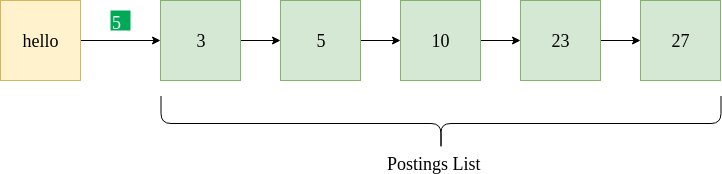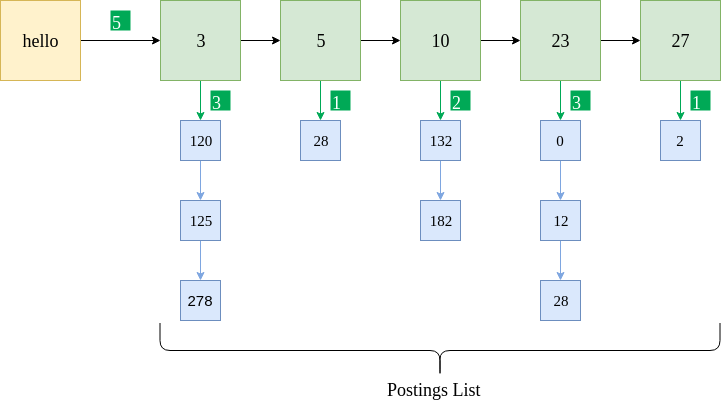# Python | Positional Index

• Last Updated : 13 Sep, 2021

This article talks about building an inverted index for an information retrieval (IR) system. However, in a real-life IR system, we not only encounter single-word queries (such as “dog”, “computer”, or “alex”) but also phrasal queries (such as “winter is coming”, “new york”, or “where is kevin”). To handle such queries, using an inverted index is not sufficient.

To understand the motivation better, consider that a user queries “saint mary school”. Now, the inverted index will provide us a list of documents containing the terms “saint”, “mary” and “school” independently. What we actually require, however, are documents where the entire phrase “saint mary school” appears verbatim. In order to successfully answer such queries, we require an index of documents that also stores the positions of terms.

Postings List
In the case of the inverted index, a postings list is a list of documents where the term appears. It is typically sorted by the document ID and stored in the form of a linked list.The above figure shows a sample postings list for the term “hello”. It indicates that “hello” appears in documents with docIDs 3, 5, 10, 23 and 27. It also specifies the document frequency 5 (highlighted in green). Given is a sample Python data format containing a dictionary and linked lists to store the postings list.

`{"hello" : [5, [3, 5, 10, 23, 27] ] }`

In the case of the positional index, the positions at which the term appears in a particular document is also stored along with the docID.The above figure shows the same postings list implemented for a positional index. The blue boxes indicate the position of the term “hello” in the corresponding documents. For instance, “hello” appears in document 5 at three positions: 120, 125, and 278. Also, the frequency of the term is stored for each document. Given is a sample Python data format for the same.

`{"hello" : [5, [ {3 : [3, [120, 125, 278]]}, {5 : [1,  ] }, {10 : [2, [132, 182]]}, {23 : [3, [0, 12, 28]]}, {27 : [1, ]} ] }`

One can also omit the term frequency in the individual documents for the sake of simplicity (as done in the sample code). The data format then looks as follows.

`{"hello" : [5, {3 : [120, 125, 278]}, {5 : }, {10 : [132, 182]}, {23 : [0, 12, 28]}, {27 : } ] }`

Steps to build a Positional Index

• Fetch the document.
• Remove stop words, stem the resulting words.
• If the word is already present in the dictionary, add the document and the corresponding positions it appears in. Else, create a new entry.
• Also update the frequency of the word for each document, as well as the no. of documents it appears in.

Code
In order to implement a positional index, we make use of a sample dataset called “20 Newsgroups”.

## Python3

 `# importing libraries` `import` `numpy as np` `import` `os` `import` `nltk` `from` `nltk.stem ``import` `PorterStemmer` `from` `nltk.tokenize ``import` `TweetTokenizer` `from` `natsort ``import` `natsorted` `import` `string`   `def` `read_file(filename):` `    ``with ``open``(filename, ``'r'``, encoding ``=``"ascii"``, errors ``=``"surrogateescape"``) as f:` `        ``stuff ``=` `f.read()`   `    ``f.close()` `    `  `    ``# Remove header and footer.` `    ``stuff ``=` `remove_header_footer(stuff)` `    `  `    ``return` `stuff`   `def` `remove_header_footer(final_string):` `    ``new_final_string ``=` `""` `    ``tokens ``=` `final_string.split(``'\n\n'``)`   `    ``# Remove tokens and tokens[-1]` `    ``for` `token ``in` `tokens[``1``:``-``1``]:` `        ``new_final_string ``+``=` `token``+``" "` `    ``return` `new_final_string`   `def` `preprocessing(final_string):` `        ``# Tokenize.` `    ``tokenizer ``=` `TweetTokenizer()` `    ``token_list ``=` `tokenizer.tokenize(final_string)`   `    ``# Remove punctuations.` `    ``table ``=` `str``.maketrans('``', '``', '``\t')` `    ``token_list ``=` `[word.translate(table) ``for` `word ``in` `token_list]` `    ``punctuations ``=` `(string.punctuation).replace(``"'"``, "")` `    ``trans_table ``=` `str``.maketrans('``', '``', punctuations)` `    ``stripped_words ``=` `[word.translate(trans_table) ``for` `word ``in` `token_list]` `    ``token_list ``=` `[``str` `for` `str` `in` `stripped_words ``if` `str``]`   `    ``# Change to lowercase.` `    ``token_list ``=``[word.lower() ``for` `word ``in` `token_list]` `    ``return` `token_list`   `# In this example, we create the positional index for only 1 folder.` `folder_names ``=` `[``"comp.graphics"``]`   `# Initialize the stemmer.` `stemmer ``=` `PorterStemmer()`   `# Initialize the file no.` `fileno ``=` `0`   `# Initialize the dictionary.` `pos_index ``=` `{}`   `# Initialize the file mapping (fileno -> file name).` `file_map ``=` `{}`   `for` `folder_name ``in` `folder_names:`   `    ``# Open files.` `    ``file_names ``=` `natsorted(os.listdir(``"20_newsgroups/"` `+` `folder_name))`   `    ``# For every file.` `    ``for` `file_name ``in` `file_names:`   `        ``# Read file contents.` `        ``stuff ``=` `read_file(``"20_newsgroups/"` `+` `folder_name ``+` `"/"` `+` `file_name)` `        `  `        ``# This is the list of words in order of the text.` `        ``# We need to preserve the order because we require positions.` `        ``# 'preprocessing' function does some basic punctuation removal,` `        ``# stopword removal etc.` `        ``final_token_list ``=` `preprocessing(stuff)`   `        ``# For position and term in the tokens.` `        ``for` `pos, term ``in` `enumerate``(final_token_list):` `            `  `                    ``# First stem the term.` `                    ``term ``=` `stemmer.stem(term)`   `                    ``# If term already exists in the positional index dictionary.` `                    ``if` `term ``in` `pos_index:` `                        `  `                        ``# Increment total freq by 1.` `                        ``pos_index[term][``0``] ``=` `pos_index[term][``0``] ``+` `1` `                        `  `                        ``# Check if the term has existed in that DocID before.` `                        ``if` `fileno ``in` `pos_index[term][``1``]:` `                            ``pos_index[term][``1``][fileno].append(pos)` `                            `  `                        ``else``:` `                            ``pos_index[term][``1``][fileno] ``=` `[pos]`   `                    ``# If term does not exist in the positional index dictionary ` `                    ``# (first encounter).` `                    ``else``:` `                        `  `                        ``# Initialize the list.` `                        ``pos_index[term] ``=` `[]` `                        ``# The total frequency is 1.` `                        ``pos_index[term].append(``1``)` `                        ``# The postings list is initially empty.` `                        ``pos_index[term].append({})      ` `                        ``# Add doc ID to postings list.` `                        ``pos_index[term][``1``][fileno] ``=` `[pos]`   `        ``# Map the file no. to the file name.` `        ``file_map[fileno] ``=` `"20_newsgroups/"` `+` `folder_name ``+` `"/"` `+` `file_name`   `        ``# Increment the file no. counter for document ID mapping              ` `        ``fileno ``+``=` `1`   `# Sample positional index to test the code.` `sample_pos_idx ``=` `pos_index[``"andrew"``]` `print``(``"Positional Index"``)` `print``(sample_pos_idx)`   `file_list ``=` `sample_pos_idx[``1``]` `print``(``"Filename, [Positions]"``)` `for` `fileno, positions ``in` `file_list.items():` `    ``print``(file_map[fileno], positions)`

Output:

```Positional Index
[10, {215: , 539: , 591: , 616: [462, 473], 680: , 691: , 714: , 809: , 979: }]
Filename, [Positions]
20_newsgroups/comp.graphics/38376 
20_newsgroups/comp.graphics/38701 
20_newsgroups/comp.graphics/38753 
20_newsgroups/comp.graphics/38778 [462, 473]
20_newsgroups/comp.graphics/38842 
20_newsgroups/comp.graphics/38853 
20_newsgroups/comp.graphics/38876 
20_newsgroups/comp.graphics/38971 
20_newsgroups/comp.graphics/39663 ```

My Personal Notes arrow_drop_up
Recommended Articles
Page :